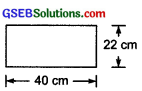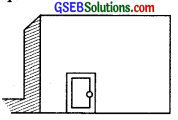# GSEB Solutions Class 7 Maths Chapter 11 Perimeter and Area Ex 11.1

Gujarat Board GSEB Textbook Solutions Class 7 Maths Chapter 11 Perimeter and Area Ex 11.1 Textbook Questions and Answers.

## Gujarat Board Textbook Solutions Class 7 Maths Chapter 11 Perimeter and Area Ex 11.1Question 1.
The length and the breadth of a rectangular piece of land are 500 m and 300 m respectively. Find:
(i) its area
(ii) the cost of the land, if 1 m² of the land costs ₹ 10,000.
Solution:
(i) Length of the rectangle (I) = 500 m
Breadth of the rectangle (b) = 300 m
∴ Area = l x b
= 500 m x 300 m = 150000 m²

(ii) ∵ Cost of 1 m² of land = ₹ 10,000
∴ Cost of 150000 m² of land
= ₹ 10000 x 150000 = ₹ 1,50,00,00,000

Question 2.
Find the area of a square park whose perimeter is 320 m.
Solution:
∵ Perimeter of the square park = 320 m
i.e. 4 x Side = 320 m
∴ Side = $$\frac { 320 }{ 4 }$$ = m = 80 m
Now, area of the square park = Side x Side = 80 m x 80 m = 6400 m²Question 3.
Find the breadth of a rectangular plot of land, if its area is 440 m² and the length is 22 m. Also find its perimeter.
Solution:
Length of the rectangle = 22 m
Suppose breadth of rectangle = b m
∴ Area of the rectangle = 22 x b
or 22 x b = 440 (∵ Area = 440 m2)
or b = $$\frac { 440 }{ 22 }$$ = 20 m
Thus, the breadth of the rectangle = 20m
Now, perimeter of a rectangle = 2(1 + b)
= 2(22 + 20) m = 2(42) m = 84 m.

Question 4.
The perimeter of a rectangular sheet is 100 cm. If the length is 35 cm, find its breadth. Also find the area.
Solution:
Perimeter of the rectangular sheet = 100 cm
Length (l) = 35 cm, Suppose breadth = b cm
∴ 2(1 + b) = 100 cm
or 2(35 + b) = 100 cm
or 35 + b = $$\frac { 100 }{ 2 }$$ cm = 50 cm
or b = (50 – 35) cm = 15 cm
∴ Breadth of the rectangular sheet =15 cm
Now, area of a rectangle = l x b
∴ Area of the rectangular sheet = 35 x 15 cm² = 525 cm²

Question 5.
The area of a square park is the same as of a rectangular park. If the side of the square park is 60 m and the length of the rectangular park is 90 m, find the breadth of the rectangular park.
Solution:
Side of the square park = 60 m
∴ Area of the square park = 60 x 60 m²
= 3600 m²
∵ [Area of the rectangular park] = [Area of the square park] 3600 m²
∵ Length of the rectangular park = 90 m
∴ Breadth of the rectangular park
= $$\frac { 3600 }{ 90 }$$ m = 40 m.Question 6.
A wire is in the shape of a rectangle. Its length is 40 cm arid breadth is 22 cm. If the same wire is rebent in the shape of a square, what will be the measure of each side? Also find which shape encloses more area?Solution:
Length (l) = 40 cm, Breadth (b) = 22 cm
∴ Perimeter = 2(l + b) = 2(40 + 22) cm
= 2(62) cm = 124 cm
∴ Area of the rectangle = l x b
= 40 cm x 22 cm = 880 cm²
∵ The same wire is rebent to form a square.
∴ Perimeter of square = Perimeter of the rectangle
∴ Perimeter of the square = 124 cm
∴ Side of the square = $$\frac { 124 }{ 4 }$$ = 31 cm
Area of the square = 31 cm x 31 cm = 961 cm²
∵ 961 cm² > 880 cm²
∴ Area of the square is more than the rectangle.

Question 7.
The perimeter of a rectangle is 130 cm. If the breadth of the rectangle is 30 cm, find its length. Also find the area of the rectangle.
Solution:
Perimeter of the rectangle = 130 cm
Breadth of the rectangle = 30 cm
Let the length of the rectangle be ‘l’.
∴ 2(1 + 30) = 130
or l + 30 = $$\frac { 130 }{ 2 }$$ = 65
or l = 65 – 30 = 35 cm
∴ Length of the rectangle = 35 cm
Now, area of the rectangle = l x b
= 35 x 30 cm² = 1050 cm²Question 8.
A door of length 2 m and breadth 1 m is fitted in a wall. The length of the wall is 4.5 m and the breadth is 3.6 m. Find the cost of white washing the wall, if the rate of white washing the wall is ₹ 20 per m².Solution:
Length of the wall (l) = 4.5 m
Breadth of the wall (b) = 3.6 m
∴ Area of the wall = l x b
= (4.5 m) x (3.6 m)
= $$\frac { 45 }{ 10 }$$ x $$\frac { 36 }{ 10 }$$
= 16.2 m²
Area of the door = 2m x 1 m = 2m²
∴ Area to be white washed
= [Area of the wall] – [Area of the door]
= 16.2 m² – 2 m² = 14.2 m²
Since, rate of white washing = ₹ 20 per m²
∴ Cost of white washing = ₹ 20 x (14.2) = ₹ 284